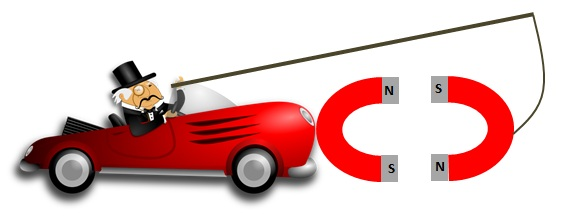# What is Newton’s Third Law of Motion

## Newton’s Third Law of Motion Definition

Newton’s Third Law of Motion says that if a body A exerts a force on body B, then the body B exerts a force of equal magnitude, in the opposite direction, on the body A.

Often, one of these forces is called “action”  and the other one “reaction”. Using these two terms, another way that people use to state Newton’s third law of motion is to say,  For every action, there is an equal and opposite reaction. However, I slightly prefer the statement I gave earlier because it also specifies that action and reaction act on two different bodies.

The two forces mentioned here are called a Newton’s third law pairs (or an action-reaction pair). Newton’s third law pairs have the following properties:

1. They are of the same type
2. They have the same magnitude
3. They act in opposite directions
4. They act along the same line
5. They act for the same duration
6. They act on two separate bodies

For example, if a person pushes a wall, the wall exerts a force of equal magnitude in the opposite direction back on the person. The person’s push on the wall is a contact force, and the wall’s push on the person is also a contact force.

## Free Body Diagrams and Newton’s Third Law of Motion

Before reading this section, make sure you’re familiar with different types of forces that come up when we make these calculations.

To illustrate forces acting on bodies, we often draw free body diagrams. In these diagrams, we draw each body involved in a given situation separately, showing only the forces that act on that body. For instance, let’s imagine an apple resting on a table.The free body diagram for the apple and the table would be as follows:In the above diagram, you can identify one Newton’s third law pair. The apple pushes down on the table ($R_{TA}$), and the table pushes back up on the apple ($R_{AT}$).

The apple is at rest, so the forces on the apple are balanced (according to Newton’s first law). This way, the upward push on the apple by the table ($R_{AT}$) is balanced by the downward pull on the apple by Earth (due to gravity) ($W_{A}$). It is important to note that these two forces are not Newton’s third law pairs. One is gravitational, the other is a normal reaction force. The weight of the apple comes from the Earth pulling down on the apple with a gravitational force. Then,  the apple pulls the Earth upwards with a gravitational force with an equal magnitude. This is the force that would form the third law pair with the apple’s weight. This force acts on the Earth and this force is not shown in the diagram.

So, any object experiencing weight is also pulling the Earth upwards with a force equal to that weight. Of course, we never see the Earth rushing up to meet the object. This is because, according to$F=ma$,$a=\frac{F}{m}$. For an upward pull on Earth that has a magnitude of a typical object’s weight, the acceleration of Earth is extremely tiny because Earth has a very large mass.

## Newton’s Third Law of Motion Example

An apple with a mass of 0.13 kg is falling. Find the weight of the apple, and the Newton’s third law pair of the apple’s weight. State on which body this second force acts, and find this object’s acceleration.

Firstly, the weight of the apple is$W=mg=0.13\times9.81=1.28\mathrm{\:N}$. The Newton’s third law pair is the apple pulling the Earth upwards. This also has the same magnitude of 1.28 N. The Earth has a mass of 5.97×1024 kg. The acceleration of Earth due to this force is$a=\frac{F}{m}=\frac{1.28}{5.97\times10^{24}}=2.13\times10^{-25}$ m s-2, which is negligibly small.

We make use of Newton’s third law of motion when we peddle a boat. With the paddle, we push water backward, and according to Newton’s third law of motion, the water pushes the paddle forward. Because the paddle is attached to the boat, the boat also moves forward with the paddle. Similarly, a rocket is also able to be launched thanks to Newton’s third law of motion. The rocket ejects a mass of air as exhaust downwards, and the air propels the rocket upward in turn.

A good example that illustrates a  failure to understand Newton’s third law of motion is the concept of a perpetually moving car powered by a pair of magnets, often shared on internet trolling sites. The idea is illustrated below:According to this idea, the magnet attached to the bonnet of the car will be forever pulled forward by the magnet held in front of it. Since the attraction continues indefinitely, the car would be accelerated forward forever.

This naive idea does not work because, according to Newton’s third law of motion, the magnet on the car bonnet will attract the magnet in front of it with an equal and opposite force (to the left, in this diagram). Since this magnet is also attached to the car by the pole, this force would cause the car to move backwards. In the end, the two forces would completely cancel each other (they are Newton’s third law pairs, so thy have the same magnitudes and opposite directions), and the car will remain stationary.